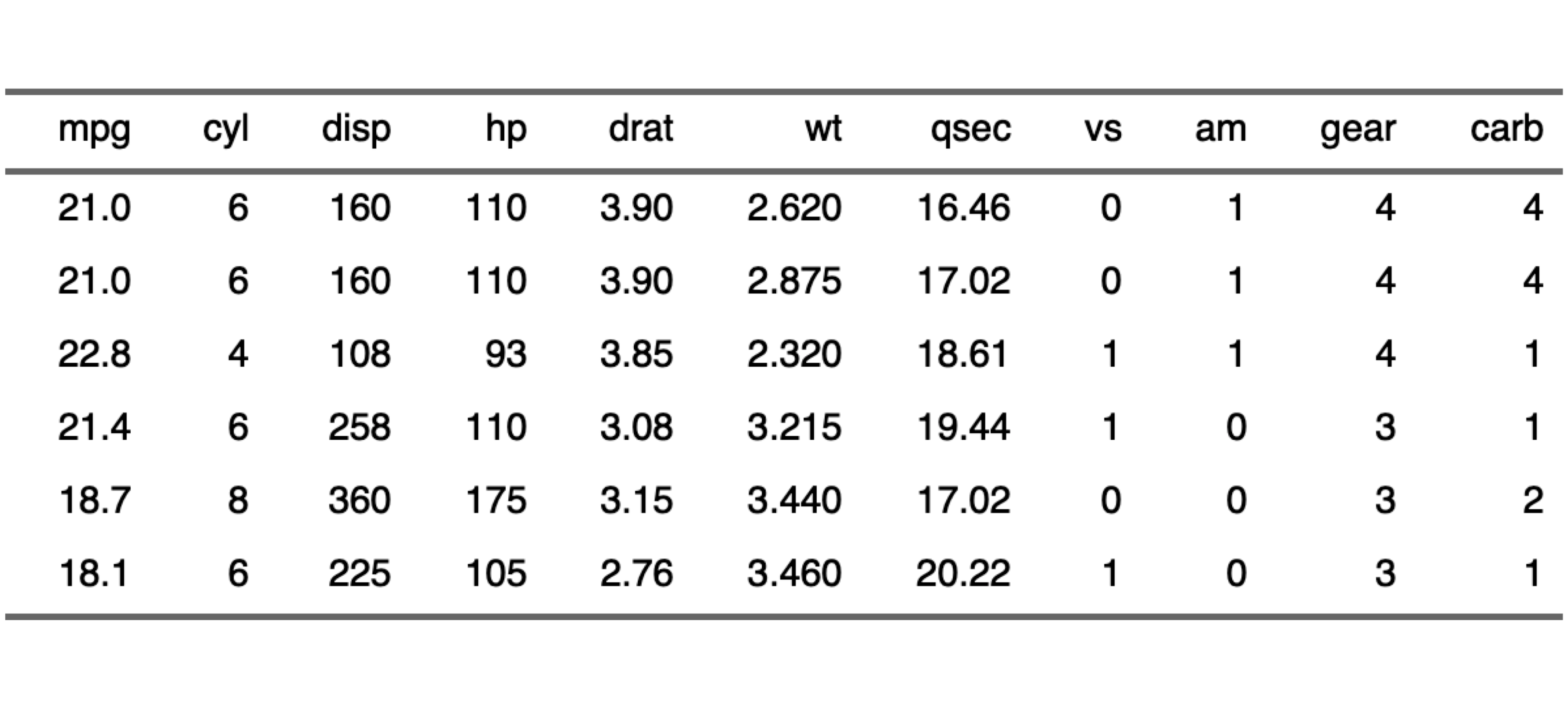Want to share your content on R-bloggers? click here if you have a blog, or here if you don't.

flextable 0.5.4 is now on CRAN.
It contains a new output option that some users were asking: image output.
You can now save a flextable as a png or pdf file with function
save_as_image.

The solution was existing since a long time but was buried in a
stackoverflow question
instead of being provided in flextable as an option.

This functionality is letting other options to be available, you can now use method
plot and
also as_raster and
do whatever you’d like with the raster (combine with a ggplot object for example).

## Demo

First, let’s create a simple flextable.

```library(flextable)
ft <- flextable( head( mtcars ) )
ft <- autofit(ft)
ft```

 mpg cyl disp hp drat wt qsec vs am gear carb 21.000 6.000 160.000 110.000 3.900 2.620 16.460 0.000 1.000 4.000 4.000 21.000 6.000 160.000 110.000 3.900 2.875 17.020 0.000 1.000 4.000 4.000 22.800 4.000 108.000 93.000 3.850 2.320 18.610 1.000 1.000 4.000 1.000 21.400 6.000 258.000 110.000 3.080 3.215 19.440 1.000 0.000 3.000 1.000 18.700 8.000 360.000 175.000 3.150 3.440 17.020 0.000 0.000 3.000 2.000 18.100 6.000 225.000 105.000 2.760 3.460 20.220 1.000 0.000 3.000 1.000

You can save it as a png:

`save_as_image(ft, path = "name.png")`

You can plot it:

`plot(ft)`Or combine the table with a ggplot object:

```library(ggplot2)
library(grid)
library(cowplot)

ft_raster <- as_raster(ft)

anyplot <- qplot(speed, dist, data = cars, geom = "point")

gflextable <- ggplot() +
theme_void() +
annotation_custom(rasterGrob(ft_raster), xmin=-Inf, xmax=Inf, ymin=-Inf, ymax=Inf)

plot_grid(anyplot, gflextable, nrow = 2, ncol = 1, rel_heights = c(4, 1) )```When a flextable is printed, it may be useful to know the exact width and height
of the table to be sure to set up the correct aspect ratio. The function
`flextable_dim()` will provide these informations without the need to produce
the image.

```dims <- flextable_dim(ft)
dims```

```#> \$widths
#>  7.948045
#>
#> \$heights
#>  2.035298
#>
#> \$aspect_ratio
#>  0.2560753```

You can reuse them as values for knitr chunk options `fig.asp`, `fig.width` and `fig.height`.